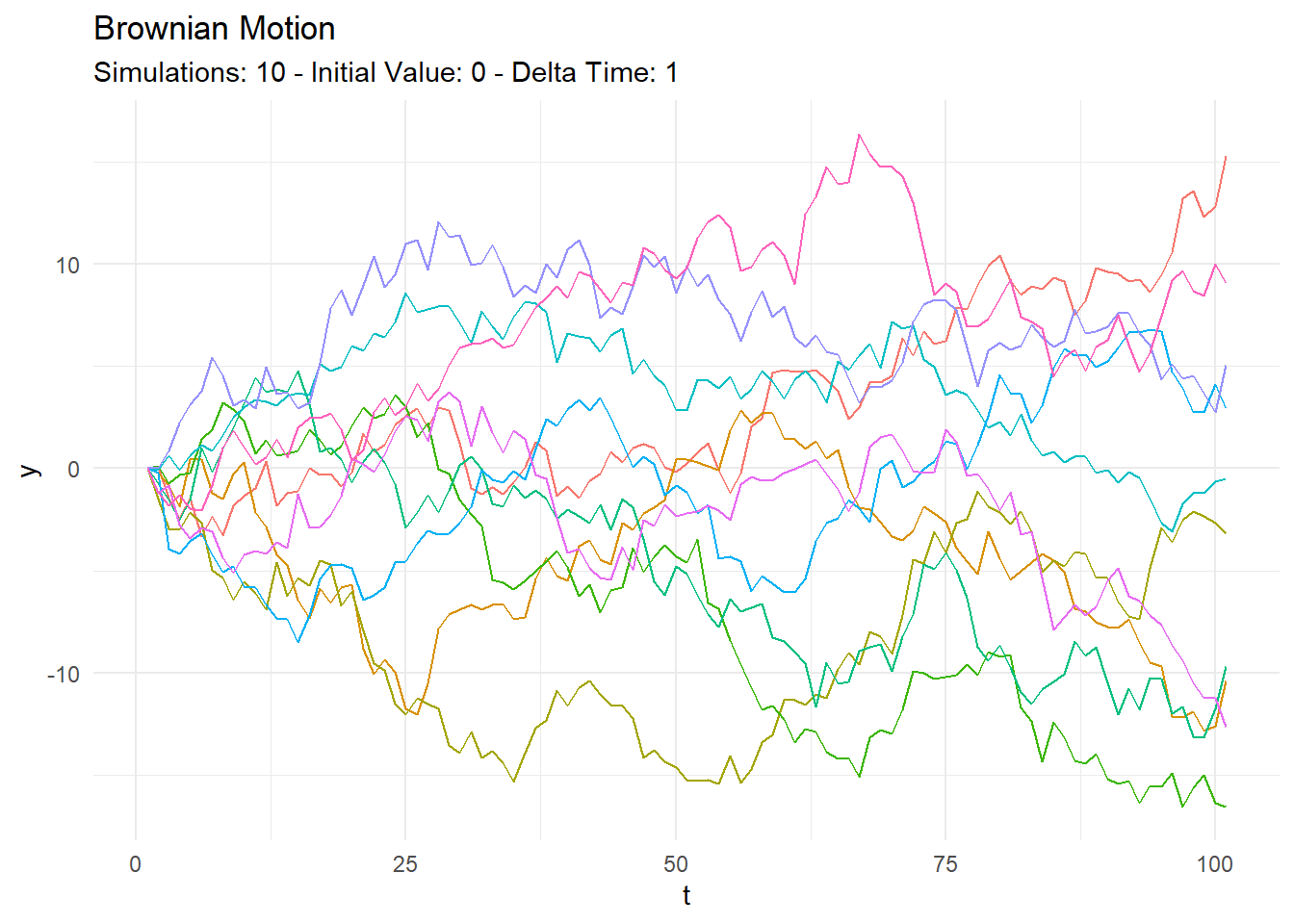Want to share your content on R-bloggers? click here if you have a blog, or here if you don't.

# Introduction

R is a powerful programming language that is widely used for data analysis, visualization, and machine learning. One of the features of R that makes it versatile and flexible is the ability to assign attributes to functions. Attributes are metadata associated with an object in R, and they can be used to store additional information about the function or to modify the behavior of the function.

In this blog post, we will discuss what attributes are, how they can be useful, and how they can be used inside R functions.

## What are Attributes in R Functions?

Attributes are pieces of information that are stored alongside an object in R. Functions are objects in R, and they can have attributes associated with them. Some of the common attributes associated with functions in R include:

1. `formals`: This attribute stores the arguments of the function and their default values.
2. `srcref`: This attribute stores the source code of the function, including the line numbers of the code.
3. `environment`: This attribute stores the environment in which the function was defined.

## How Attributes can be Useful in R Functions

Attributes can be useful in R functions in several ways, including:

1. Debugging: Attributes can be used to store information that can be used to debug functions. For example, the srcref attribute can be used to retrieve the source code of the function and the line numbers of the code, which can be useful when trying to identify the source of an error.
2. Metadata: Attributes can be used to store metadata about the function, such as the author, version, and date of creation. This information can be used to keep track of the function and to provide information about its purpose and usage.
3. Modifying Function Behavior: Attributes can be used to modify the behavior of the function. For example, the environment attribute can be used to set the environment in which the function is executed. This can be useful when creating closures or when using functions in a specific context.

## How to Use Attributes in R Functions

To access or modify the attributes of a function in R, you can use the attributes() function. For example, to retrieve the formals attribute of a function, you can use the following code:

```f <- function(x, y) { x + y }
attributes(f)```
```\$srcref
function(x, y) { x + y }```
`formals(f)`
```\$x

\$y```

To add an attribute to a function, you can use the attr() function. For example, to add a version attribute to a function, you can use the following code:

```f <- function(x, y) { x + y }
attr(f, "version") <- "1.0"
attributes(f)```
```\$srcref
function(x, y) { x + y }

\$version
 "1.0"```

To remove an attribute from a function, you can use the attributes() function with the NULL value. For example, to remove the version attribute from a function, you can use the following code:

```f <- function(x, y) { x + y }
attr(f, "version") <- "1.0"
attributes(f)\$version <- NULL
attributes(f)```
```\$srcref
function(x, y) { x + y }```

Conclusion

Attributes are a useful feature in R functions that can be used to store additional information about the function, to debug the function, and to modify its behavior. By using attributes, you can make your functions more versatile, flexible, and easier to work with.

# Function

Here is a function from my `{healthyR.ts}` package that makes use of attributes that come in from the output of another function. The function is `ts_brownian_motion_plot()`. Let’s go ahead and take a look at how the function works.

```# ...

# Attributes
atb <- attributes(.data)

# ...

ggplot2::labs(
title = atb\$.motion_type,
subtitle = paste0("Simulations: ", atb\$.num_sims,
" - Initial Value: ", round(atb\$.initial_value, 2),
" - Delta Time: ", round(atb\$.delta_time, 2))
) +
ggplot2::theme(legend.position = if(atb\$.num_sims > 9) {"none"})```

So what’s happening here is that I am taking the attributes from the incoming data which is the result of a `{healthyR.ts}` brownian motion function and setting them equal to a variable `atb`, later in the function I take that `atb` variable and pluck out certain items I want from it.

# Example

Let’s see an example in action.

```# install.packages("healthyR.ts")
library(healthyR.ts)

df <- ts_brownian_motion()

atb <- attributes(df)

atb[!names(atb) %in% "row.names"]```
```\$names
 "sim_number" "t"          "y"

\$class
 "tbl_df"     "tbl"        "data.frame"

\$.time
 100

\$.num_sims
 10

\$.delta_time
 1

\$.initial_value
 0

\$.return_tibble
 TRUE

\$.motion_type
 "Brownian Motion"```

Now to see the plat in action.

`ts_brownian_motion_plot(df, t, y)`Voila!